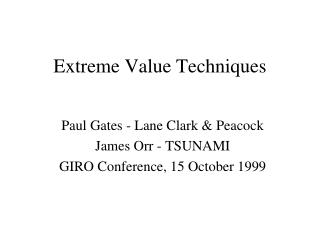DownloadDownload PresentationExtreme Value Techniques

# Extreme Value Techniques

Télécharger la présentation## Extreme Value Techniques

- - - - - - - - - - - - - - - - - - - - - - - - - - - E N D - - - - - - - - - - - - - - - - - - - - - - - - - - -
##### Presentation Transcript

1. Extreme Value Techniques Paul Gates - Lane Clark & Peacock James Orr - TSUNAMI GIRO Conference, 15 October 1999

2. Extreme Value Statistics • Motivation and Maxima Theory • Dutch Dike Problem • Threshold Theory • Motor XL Rating Problem • Questions

3. Extreme Value Statistics • “Statistical Study of Extreme Levels of a Process” • Relevant? • Catastrophic Events • Increasing Insurance Deductibles • Estimated/Probable Maximum Loss • Excess of Loss Reinsurance • Value at Risk/EPD  Capital Allocation Er…YES!

4. Hierarchy of Analysis • Experience Rating - “Burning Cost” • Central Limit Theorem • Actuaries have Added Value • Statistical Rating - “Curve Fitting” • Lognormal/Pareto (apply threshold?) • What Data do we Use?

5. A Break from “The Norm” • Central Limit Theorem • Class Average I.Q.s • Average Observations ~ N(,) • Price Insurance by “Expected Claims” • Extremal Types Theorem or Maxima Theory • Class Maximum I.Q.s • Maximum Observations ~ GEV(,,) • Price Insurance by “Expected Claims Attaching”

6. Generalized Extreme ValueDistribution Pr( X  x ) = G(x)= exp [ - (1 + ( (x-) /  ))-1/ ]

7. EVTs in Action • Netherlands Flood • De Haan - “Fighting the Arch-enemy with Mathematics”

8. Outline of Problem • Height of sea defences • 1953 storm surge - sea dike failure • Need to recommend an appropriate sea defence level • Flood probability reduced to small “p” • 1/10,000 to 1/100,000 • Extrapolation required

9. Data Analysis • High tide water levels at 5 stations • 60 to 110 years of info • “Set up” values • observed high tide level minus predicted high tide level • eliminate set up values below a threshold • utilise winter observations only • selection to achieve independence • 15 observations per year • Exceedance prob for 1953 storm = 1 / 382

10. Probability Theory • Distribution function F • X1 to Xn are independent observations • Find xp so that F(xp) = 1 - p • Lim Fr (arx+br) = lim (P ((max(X1..Xr)-br/)ar)<x) = G(x) • G(x) = exp (-(1+ x)-1/) • Generalized Extreme Value Distribution

11. Estimation Procedures • Largest observations per winter • iid observations • G(x) formula as above • maximum likelihood • Assume all set up levels above threshold Ln follow distribution 1-(1+yx/a(Ln))-1/y • Find y and a(Ln) by maximum likelihood • Generalised Pareto distribution • Combine with astronomical (predicted) levels to get absolute sea levels

12. Mr De Haan’s short cut • Lim (U(tx)-U(t))/(U(tz)-U(t)) = (x-1)/(z-1) • where U = (1/(1-F)) - return period function • high quantiles can be expressed in terms of lower quantiles • Estimation of  • Lim (U(2t)-U(t))/(U(t)-U(t/2)) = 2

13. A quick example • Find x and return period for 5m high tide

14. A quick solution • (U(2t) - U(t)) / (U(t) - U(t/2) = (30 - 10) / (10 - 4) = 2x • Whence x = 1.74 • (U(5t) - U(t)) / (U(2t) - U(t)) = (d - 10) / (30- 10) = (5x-1) / (2x-1) • Whence return period = 142 years

15. Output • Level with exceedance probability 1/10,000 • Xp • Estimate of x • x = 0 • Xp= 5.1m + sea level (reference level) • 16 foot walls required!

16. Back to James…

17. Threshold Theory • Why just use Maxima Data? • Exceedance Over Threshold: • Generalised Pareto Distribution...…but Need to Choose Threshold • Diagnostic Tool: • If GPD holds, the Mean Excess Plot……is Linear for High Values of the Threshold

18. Generalised Pareto Distribution Pr(Y  y) 1 - Pr(Y > u) [ 1 + (y-u) / ]+-1/=1 - u [ 1 + (y-u) / ]+-1/

19. Motor XL Pricing Problem • Issue - rating high XL layers • Paucity of data • eg £10m xs £10m • largest single claim = £9.2m (BIWP 1999) • Extrapolating from data • Use data over certain threshold • Fit curve (GPD)

20. Analysis • Claims from 1985 - 1998 (146) • Paid & Reported Losses • Data Above Threshold • Current / Largest Ever • Revaluation • Changing Exposure • Claims by Band & Development of Losses

21. Excel Output

22. Problems, Problems, Problems... • One-off events • Ogden, Woolf, Baremo • Events that haven’t happened • Tokyo earthquake,Year 2000, UK flood • Extreme Events • 2*90A, \$50Bn US earthquake, US wind • Man-made Trends • building on flood plains, global warming

23. Extreme Value Statistics Questions